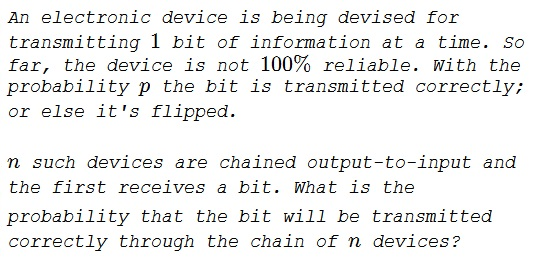# Artificially Unintelligent

### Problem### Solution 1

Number of bit flips has to be even. Let $q=1-p$.

$\displaystyle P=\sum_{\{k|k\equiv 0~(mod~2),k\leq n\}} C(n,k)q^kp^{n-k}$

Using the binomial expansion

$\displaystyle P= \begin{cases} \displaystyle \frac{(q+p)^n+(q-p)^n}{2}=\frac{1+(1-2p)^n}{2},~n~\text{even} \\ \displaystyle \frac{(q+p)^n-(q-p)^n}{2}=\frac{1-(1-2p)^n}{2},~n~\text{odd} \end{cases}$

Since, for odd $n,$ $(-1)^n=-1,$ $\displaystyle \frac{1+(2p-1)^n}{2}$ so that we can combine the two expressions into one:

$\displaystyle \frac{1+(2p-1)^n}{2}.$

### Solution 2

Let $\displaystyle \left(\begin{array}\,1\\0\end{array}\right)$ represent the correct bit, and $\displaystyle \left(\begin{array}\,0\\1\end{array}\right)$ the flipped bit. The transition probabilities between two states are given by the (diagonalizable) transfer matrix:

$\displaystyle T=\left(\begin{array}{cc}p&1-p\\1-p&p \end{array}\right)=X^{-1}\left(\begin{array}{cc}1&0\\0&2p-1\end{array}\right)X,$

where $\displaystyle X=\frac{1}{\sqrt{2}}\left(\begin{array}{cr}\,1&-1\\1&1\end{array}\right)$ and $\displaystyle X=\frac{1}{\sqrt{2}}\left(\begin{array}{rc}\,1&1\\-1&1\end{array}\right).$ For $n$ transmissions, the matrix is:

$\displaystyle T^n=X^{-1}\left(\begin{array}{cc}1&0\\0&(2p-1)^n\end{array}\right)X=\frac{1}{2}\left(\begin{array}{cc}1+(2p-1)^n&1-(2p-1)^n\\1-(2p-1)^n&1+(2p-1)^n\end{array}\right).$

The probability of measuring the correct bit after $n$ transmission is $\displaystyle (T^n)_{11}=\frac{1}{2}(1+(2p-1)^n).$

### Solution 3

Using the binomial theorem $\displaystyle (a+b)^n=\sum_{k=0}^n{n\choose k}a^{n-k}b^k,$ we want only the terms with an even number of failures:

\displaystyle \begin{align} P&=\sum_{k=0}^n{n\choose k}p^{n-k}(1-p)^k\cdot\frac{1+(-1)^k}{2}\\ &=\frac{1}{2}\left(\sum_{k=0}^n{n\choose k}p^{n-k}(1-p)^k\right)+\frac{1}{2}\left(\sum_{k=0}^n{n\choose k}p^{n-k}(p-1)^k\right)\\ &=\frac{1}{2}\left((p+(1-p))^n+(2p-1)^n\right)\\ &=\frac{1}{2}+\frac{1}{2}(2p-1)^n. \end{align}

### Solution 4

We need either $0$ or an even number of switches for transmission to be correct. Let $\displaystyle \phi=p^x{n\choose x}(1-p)n-x$ be the pdf of a binomial distribution $N(n,p)$ with $n$ trials and the probability of success $p,$ for $x$ successes. We need $x$ to be even, $x=0,2,\ldots,n.$

The probability under consideration is

\displaystyle \begin{align} P(n)&=\sum_{x=0}^{n/2}p^{2x}{n\choose 2x}(1-p)^{n-2x}=\frac{1}{2}\left(1+(2p-1)^n\right)\\ &\approx\frac{1}{2}, \end{align}

for large values of $n.$ And, since $0\le p\lt 1,$

$\displaystyle \lim_{n\to\infty}\frac{1}{2}\left(1+(2p-1)^n\right)=\frac{1}{2}.$

### Solution 5

Let $P(n,1)$ be the probability that the message gets transmitted correctly given that it is correct and it has to be transmitted $n$ more times. Let $P(n,0)$ be the probability that the message gets transmitted correctly given that it is incorrect and it has to be transmitted $n$ more times. Recursively we can compute these probabilities as

\begin{align}P(n,1) &= p P(n-1,1) + (1-p) P(n-1,0)\\ P(n,0) &= p P(n-1,0) + (1-p) P(n-1,1) \end{align}

With boundary conditions

$P(0,1) = 1\\ P(0,0) = 0$

From the previous equations we can show that

$P(n,1) + P(n,0) = P(n-1,1)+P(n-1,0)$

which implies that

$P(n,0) = 1-P(n,1)$

Substite into the first equation and solve to get

\displaystyle \begin{align}P(n,1) &= (2p-1)P(n-1,1)+1-p\\ P(n,1) &= (2p-1)^{n} + (1 - p) \sum\limits_{i=0}^{n-1}(2p-1)^{i}\\ P(n,1) &= (2p-1)^{n} + (1 - p) \frac{1-(2p-1)^n}{1-(2p-1)}\\ P(n,1) &= \frac{(2p-1)^n}{2} + \frac{1}{2} \end{align}

### Remark

As $n\to\infty,$ the probability tends to $\displaystyle \frac{1}{2},$ regardless of $p.$

### Acknowledgment

This is a paraphrase of a problem from P. Nahin's Will You Be Alive In 10 Years From Now? (Princeton University Press, 2013, Ch 11).

Solution 1 is by Amit Itagi; Solution 2 is by Hélvio Vairinhos; Solution 3 is by Timon Knigge; Solution 4 is by N. N. Taleb; Solution 5 is by Alejandro Rodríguez.